### Home > PC > Chapter 2 > Lesson 2.3.2 > Problem2-91

2-91.
1. Evaluate each expression. Homework Help ✎1.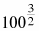2.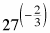3.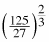4.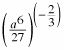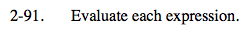When possible, take the root first and then the power.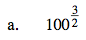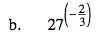$\text{Flip the fraction first to }\frac{1}{27} \text{ eliminating the negative exponent.}$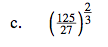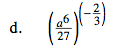$\Big(\frac{27}{a^6}\Big)^{2/3}$